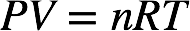# Problem: A gas sample at STP contains 1.26 g oxygen and 1.76 g nitrogen.What is the volume of the gas sample?

###### FREE Expert Solution

The ideal gas law is as follows:At conditions of standard temperature and pressure (STP), temperature = 273.15 K and pressure = 1 atm.

We first need to determine the number of moles of O2 and N2. The molar mass of O2 is 2(16.00 g/mol O) = 32.00 g/mol and the molar mass of N2 is 2(14.01 g/mol N) = 28.02 g/mol.

82% (288 ratings)###### Problem Details

A gas sample at STP contains 1.26 g oxygen and 1.76 g nitrogen.

What is the volume of the gas sample?

Frequently Asked Questions

What scientific concept do you need to know in order to solve this problem?

Our tutors have indicated that to solve this problem you will need to apply the Standard Temperature and Pressure concept. You can view video lessons to learn Standard Temperature and Pressure. Or if you need more Standard Temperature and Pressure practice, you can also practice Standard Temperature and Pressure practice problems.